# 1. GENERAL INFORMATION### MATLAB is a very powerful package which allows to manipulate simultaneously: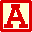VECTORS and MATRICES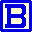COMPLEX FUNCTIONS of COMPLEX VARIABLES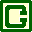DENSITY PLOTS and 3D PLOTS thanks to pcolor and mesh instructions. Moreover it allows recursion in calculating functions. Therefore it proves to be an ideal tool to study FRACTALS generated by iteration of COMPLEX VARIABLES FUNCTIONS, like MANDELBROT and JULIA sets.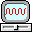THE HARDWARE ENVIRONMENT. The images we present have been generated by MATLAB starting from SCRIPTS of few rows on a Sun Spark 10 workstation.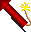THE SPEED OF GENERATION Each image takes only FEW MINUTES of machine time to be generated, since MATLAB works simultaneously with the all the elements of the matrix involving the coordinates of the pixels which is created by the function meshgrid.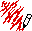PLOTS AND SCRIPTS Each plot is presented in the form of a clickable image and it is accompanied by the text of the MATLAB SCRIPT by which it has been generated.# INDEXFRACTAL GALLERY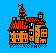CIRAM HOME PAGE